x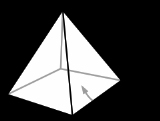Pyramid (geometry)Overview

Pyramid (disambiguation)
A pyramid is a shape with typically three or four triangular sides.Pyramid may also refer to:-Anatomy and medicine:* Petrous part of the temporal bone, the pyramid* Pyramid , the anterior part of medulla oblongata-Games and sport:...

.

In geometry
Geometry
Geometry arose as the field of knowledge dealing with spatial relationships. Geometry was one of the two fields of pre-modern mathematics, the other being the study of numbers ....

, a pyramid is a polyhedron
Polyhedron
In elementary geometry a polyhedron is a geometric solid in three dimensions with flat faces and straight edges...

formed by connecting a polygon
Polygon
In geometry a polygon is a flat shape consisting of straight lines that are joined to form a closed chain orcircuit.A polygon is traditionally a plane figure that is bounded by a closed path, composed of a finite sequence of straight line segments...

al base and a point, called the apex
Apex (geometry)
In geometry, an apex is the vertex which is in some sense the highest of the figure to which it belongs.*In an isosceles triangle, the apex is the vertex where the two sides of equal length meet, opposite the unequal third side....

. Each base edge and apex form a triangle. It is a conic solid with polygonal base.

A pyramid with an n-sided base will have n + 1 vertices, n + 1 faces, and 2n edges.Are the lateral faces congruent in a regular pyramid?Encyclopedia
Set of pyramids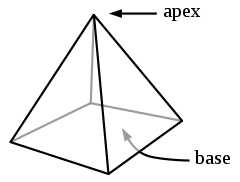Faces k triangle
Triangle
A triangle is one of the basic shapes of geometry: a polygon with three corners or vertices and three sides or edges which are line segments. A triangle with vertices A, B, and C is denoted ....

s,
1 n-gon
Polygon
In geometry a polygon is a flat shape consisting of straight lines that are joined to form a closed chain orcircuit.A polygon is traditionally a plane figure that is bounded by a closed path, composed of a finite sequence of straight line segments...

Edges 2n
Vertices n + 1
Symmetry group Cnv, [n], (*nn)
Dual polyhedron
Dual polyhedron
In geometry, polyhedra are associated into pairs called duals, where the vertices of one correspond to the faces of the other. The dual of the dual is the original polyhedron. The dual of a polyhedron with equivalent vertices is one with equivalent faces, and of one with equivalent edges is another...

Self-dual
Properties convex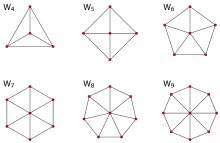Pyramid (disambiguation)
A pyramid is a shape with typically three or four triangular sides.Pyramid may also refer to:-Anatomy and medicine:* Petrous part of the temporal bone, the pyramid* Pyramid , the anterior part of medulla oblongata-Games and sport:...

.

In geometry
Geometry
Geometry arose as the field of knowledge dealing with spatial relationships. Geometry was one of the two fields of pre-modern mathematics, the other being the study of numbers ....

, a pyramid is a polyhedron
Polyhedron
In elementary geometry a polyhedron is a geometric solid in three dimensions with flat faces and straight edges...

formed by connecting a polygon
Polygon
In geometry a polygon is a flat shape consisting of straight lines that are joined to form a closed chain orcircuit.A polygon is traditionally a plane figure that is bounded by a closed path, composed of a finite sequence of straight line segments...

al base and a point, called the apex
Apex (geometry)
In geometry, an apex is the vertex which is in some sense the highest of the figure to which it belongs.*In an isosceles triangle, the apex is the vertex where the two sides of equal length meet, opposite the unequal third side....

. Each base edge and apex form a triangle. It is a conic solid with polygonal base.

A pyramid with an n-sided base will have n + 1 vertices, n + 1 faces, and 2n edges. All pyramids are self-dual.

When unspecified, the base is usually assumed to be square.

If the base is a regular polygon
Regular polygon
A regular polygon is a polygon that is equiangular and equilateral . Regular polygons may be convex or star.-General properties:...

and the apex is above the center of the polygon, an n-gonal pyramid will have Cnv symmetry.

Pyramids are a subclass of the prismatoid
Prismatoid
In geometry, a prismatoid is a polyhedron where all vertices lie in two parallel planes....

s.

## Pyramids with regular polygon faces

The regular
Regular polyhedron
A regular polyhedron is a polyhedron whose faces are congruent regular polygons which are assembled in the same way around each vertex. A regular polyhedron is highly symmetrical, being all of edge-transitive, vertex-transitive and face-transitive - i.e. it is transitive on its flags...

tetrahedron
Tetrahedron
In geometry, a tetrahedron is a polyhedron composed of four triangular faces, three of which meet at each vertex. A regular tetrahedron is one in which the four triangles are regular, or "equilateral", and is one of the Platonic solids...

, one of the Platonic solid
Platonic solid
In geometry, a Platonic solid is a convex polyhedron that is regular, in the sense of a regular polygon. Specifically, the faces of a Platonic solid are congruent regular polygons, with the same number of faces meeting at each vertex; thus, all its edges are congruent, as are its vertices and...

s, is a triangular pyramid all of whose faces are equilateral triangles. Besides the triangular pyramid, only the square and pentagonal pyramids can be composed of regular convex polygons, in which case they are Johnson solid
Johnson solid
In geometry, a Johnson solid is a strictly convex polyhedron, each face of which is a regular polygon, but which is not uniform, i.e., not a Platonic solid, Archimedean solid, prism or antiprism. There is no requirement that each face must be the same polygon, or that the same polygons join around...

s.
Tetrahedron
Tetrahedron
In geometry, a tetrahedron is a polyhedron composed of four triangular faces, three of which meet at each vertex. A regular tetrahedron is one in which the four triangles are regular, or "equilateral", and is one of the Platonic solids...

Square pyramid
Square pyramid
In geometry, a square pyramid is a pyramid having a square base. If the apex is perpendicularly above the center of the square, it will have C4v symmetry.- Johnson solid :...

Pentagonal pyramid
Pentagonal pyramid
In geometry, a pentagonal pyramid is a pyramid with a pentagonal base upon which are erected five triangular faces that meet at a point . Like any pyramid, it is self-dual....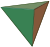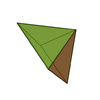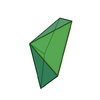### Star pyramids

Pyramids with regular star polygon bases are called star pyramids. For example, the pentagrammic pyramid has a pentagram
Pentagram
A pentagram is the shape of a five-pointed star drawn with five straight strokes...

base and 5 intersecting triangle sides.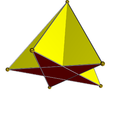## Volume

The volume
Volume
Volume is the quantity of three-dimensional space enclosed by some closed boundary, for example, the space that a substance or shape occupies or contains....

of a pyramid is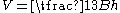where B is the area
Area
Area is a quantity that expresses the extent of a two-dimensional surface or shape in the plane. Area can be understood as the amount of material with a given thickness that would be necessary to fashion a model of the shape, or the amount of paint necessary to cover the surface with a single coat...

of the base and h the height from the base to the apex. This works for any location of the apex, provided that h is measured as the perpendicular
Perpendicular
In geometry, two lines or planes are considered perpendicular to each other if they form congruent adjacent angles . The term may be used as a noun or adjective...

distance from the plane which contains the base. In 499 AD Aryabhata
Aryabhata
Aryabhata was the first in the line of great mathematician-astronomers from the classical age of Indian mathematics and Indian astronomy...

, a mathematician
Mathematician
A mathematician is a person whose primary area of study is the field of mathematics. Mathematicians are concerned with quantity, structure, space, and change....

-astronomer
Astronomer
An astronomer is a scientist who studies celestial bodies such as planets, stars and galaxies.Historically, astronomy was more concerned with the classification and description of phenomena in the sky, while astrophysics attempted to explain these phenomena and the differences between them using...

from the classical age of Indian mathematics
Indian mathematics
Indian mathematics emerged in the Indian subcontinent from 1200 BCE until the end of the 18th century. In the classical period of Indian mathematics , important contributions were made by scholars like Aryabhata, Brahmagupta, and Bhaskara II. The decimal number system in use today was first...

and Indian astronomy, used this method in the Aryabhatiya
Aryabhatiya
Āryabhaṭīya or Āryabhaṭīyaṃ, a Sanskrit astronomical treatise, is the magnum opus and only extant work of the 5th century Indian mathematician, Āryabhaṭa.- Structure and style:...

(section 2.6)
.

The formula can be formally proved using calculus: By similarity, the dimensions of a cross section parallel to the base increase linearly from the apex to the base. Then, the cross section at any height y is the base scaled by a factor of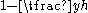, where h is the height from the base to the apex. Since the area of any shape is multiplied by the square of the shape's scaling
Scaling (geometry)
In Euclidean geometry, uniform scaling is a linear transformation that enlarges or shrinks objects by a scale factor that is the same in all directions. The result of uniform scaling is similar to the original...

factor, the area of a cross section at height y is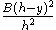. The volume is given by the integral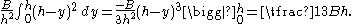The volume of a pyramid whose base is an n-sided regular polygon
Regular polygon
A regular polygon is a polygon that is equiangular and equilateral . Regular polygons may be convex or star.-General properties:...

with side length s and whose height is h is therefore: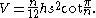The volume of a pyramid whose base is a regular n-sided polygon
Polygon
In geometry a polygon is a flat shape consisting of straight lines that are joined to form a closed chain orcircuit.A polygon is traditionally a plane figure that is bounded by a closed path, composed of a finite sequence of straight line segments...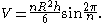The same equation,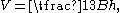also holds for cones with any base (not necessarily a polyhedron). This can be proven by the same argument as above, or, for sufficiently simple cones, by approximating the cone by pyramids; see volume of a cone.

## Surface area

The surface area
Surface area
Surface area is the measure of how much exposed area a solid object has, expressed in square units. Mathematical description of the surface area is considerably more involved than the definition of arc length of a curve. For polyhedra the surface area is the sum of the areas of its faces...

of a pyramid is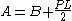where B is the base area, P is the base perimeter
Perimeter
A perimeter is a path that surrounds an area. The word comes from the Greek peri and meter . The term may be used either for the path or its length - it can be thought of as the length of the outline of a shape. The perimeter of a circular area is called circumference.- Practical uses :Calculating...

and L is the slant height: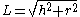where h is the pyramid altitude and r is the inradius of the base.

• Bipyramid
Bipyramid
An n-gonal bipyramid or dipyramid is a polyhedron formed by joining an n-gonal pyramid and its mirror image base-to-base.The referenced n-gon in the name of the bipyramids is not an external face but an internal one, existing on the primary symmetry plane which connects the two pyramid halves.The...

• Cone (geometry)
Cone (geometry)
A cone is an n-dimensional geometric shape that tapers smoothly from a base to a point called the apex or vertex. Formally, it is the solid figure formed by the locus of all straight line segments that join the apex to the base...

• Trigonal pyramid (chemistry)
Trigonal pyramid (chemistry)
In chemistry, a trigonal pyramid is a molecular geometry with one atom at the apex and three atoms at the corners of a trigonal base. When all three atoms at the corners are identical, the molecule belongs to point group C3v. One example of a molecule with a trigonal pyramidal geometry is ammonia...

• Frustum
Frustum
In geometry, a frustum is the portion of a solid that lies between two parallel planes cutting it....